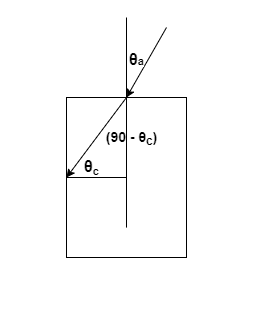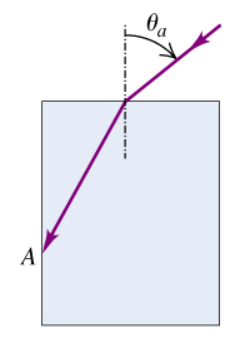# Problem: A ray of light is incident in air on a block of a transparent solid whose index of refraction is n. If n = 1.34, what is the largest angle of incidence for which total internal reflection will occur at the vertical face (point shown in the figure )?

###### FREE Expert Solution

The critical angle is expressed as:

$\overline{){{\mathbf{\theta }}}_{{\mathbf{c}}}{\mathbf{=}}{\mathbf{s}}{\mathbf{i}}{{\mathbf{n}}}^{\mathbf{-}\mathbf{1}}{\mathbf{\left(}}\frac{{\mathbf{\eta }}_{\mathbf{a}}}{{\mathbf{\eta }}_{\mathbf{c}}}{\mathbf{\right)}}}$

Snell's law:

$\overline{){{\mathbf{\eta }}}_{{\mathbf{1}}}{\mathbf{s}}{\mathbf{i}}{\mathbf{n}}{{\mathbf{\theta }}}_{{\mathbf{1}}}{\mathbf{=}}{{\mathbf{\eta }}}_{{\mathbf{2}}}{\mathbf{s}}{\mathbf{i}}{\mathbf{n}}{{\mathbf{\theta }}}_{{\mathbf{2}}}}$

This can be expressed in the diagram below;θc = sin-1(1/1.34) = 48.2°

94% (250 ratings)###### Problem Details

A ray of light is incident in air on a block of a transparent solid whose index of refraction is n. If n = 1.34, what is the largest angle of incidence for which total internal reflection will occur at the vertical face (point shown in the figure )?